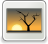# Calculation of hybrid multi-layered perceptron neural network output using matrix multiplication

Siti Maryam, Sharun and Mohd Yusoff, Mashor and Ehkan, Phaklen and Lee, Yee Ann (2017) Calculation of hybrid multi-layered perceptron neural network output using matrix multiplication. In: 3rd International Conference on Electronic Design, ICED 2016, 11- 12 August 2015, Phuket; Thailand.Image FH03-FRIT-17-08229.jpg Restricted to Registered users only Download (192kB)

## Abstract

After some studies on the HMLP neural network output equation, it was found out that parts of the equation resemble matrix multiplication operation. Therefore, an approach to calculate the output equation of the HMLP using matrix multiplication method was proposed. The proposed approach was simulated and compared with another approach to calculate HMLP output using loops. The result proved that the output of the HMLP calculated using matrix multiplication method is the same as when being calculated using looping method. When comparing the processing time of both methods, the matrix multiplication method is faster than looping method for HMLP with more nodes. However, looping method calculated the output faster for HMLP with less nodes. This paper presents part of an ongoing study with the goal to develop an architecture for implementing the HMLP on FPGA

Item Type: Conference or Workshop Item (Paper) T Technology > TK Electrical engineering. Electronics Nuclear engineering Faculty of Innovative Design & Technology Muhammad Akmal Azhar 19 Nov 2020 07:18 19 Nov 2020 07:18 http://eprints.unisza.edu.my/id/eprint/1682View Item